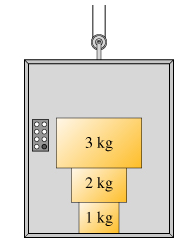# Problem: Three blocks are stacked on top of each other inside an elevator as shown in the figure.A. Answer the following questions with reference to the eight forces defined as follows.the force of the 3 kg block on the 2 kg block, F3 on 2,the force of the 2 kg block on the 3 kg block, F2 on 3,the force of the 3 kg block on the 1 kg block, F3 on 1,the force of the 1 kg block on the 3 kg block, F1 on 3,the force of the 2 kg block on the 1 kg block, F2 on 1,the force of the 1 kg block on the 2 kg block, F1 on 2,the force of the 1 kg block on the floor, F1 on the floor, andthe force of the floor on the 1 kg block, Ffloor on 1.B. Assume the elevator is at rest. Rank the magnitude of the forces.Rank from largest to smallest. To rank items as equivalent, overlap them.

###### FREE Expert Solution

For the arrangement, F = mg

91% (195 ratings)###### Problem Details

Three blocks are stacked on top of each other inside an elevator as shown in the figure.

A. Answer the following questions with reference to the eight forces defined as follows.

1. the force of the 3 kg block on the 2 kg block, F3 on 2,
2. the force of the 2 kg block on the 3 kg block, F2 on 3,
3. the force of the 3 kg block on the 1 kg block, F3 on 1,
4. the force of the 1 kg block on the 3 kg block, F1 on 3,
5. the force of the 2 kg block on the 1 kg block, F2 on 1,
6. the force of the 1 kg block on the 2 kg block, F1 on 2,
7. the force of the 1 kg block on the floor, F1 on the floor, and
8. the force of the floor on the 1 kg block, Ffloor on 1.B. Assume the elevator is at rest. Rank the magnitude of the forces.

Rank from largest to smallest. To rank items as equivalent, overlap them.